EKaye

•Tessellation 2

Book

EKaye

•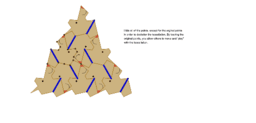Rotation Tessellation Triangle Step 21

Activity

EKaye

•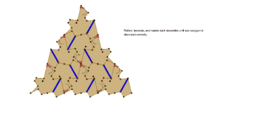Rotation Tessellation Triangle Step 20

Activity

EKaye

•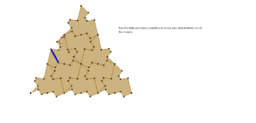Rotation Tessellation Triangle Step 19

Activity

EKaye

•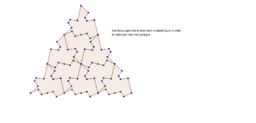Rotation Tessellation Triangle Step 18

Activity

EKaye

•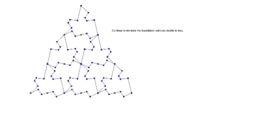Rotation Tessellation Triangle Step 17

Activity

EKaye

•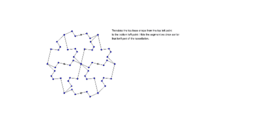Rotation Tessellation Triangle Step 16

Activity

EKaye

•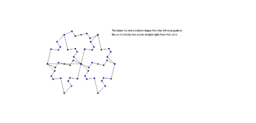Rotation Tessellation Triangle Step 15

Activity

EKaye

•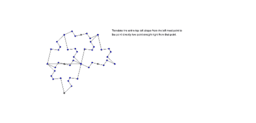Rotation Tessellation Triangle Step 14

Activity

EKaye

•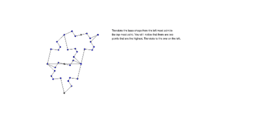Rotation Tessellation Triangle Step 13

Activity

EKaye

•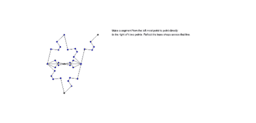Rotation Tessellation Triangle Step 12

Activity

EKaye

•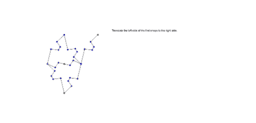Rotation Tessellation Triangle Step 11

Activity

EKaye

•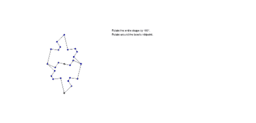Rotation Tessellation Triangle Step 10

Activity

EKaye

•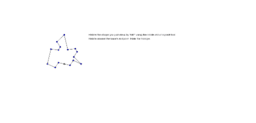Rotation Tessellation Triangle Step 9

Activity

EKaye

•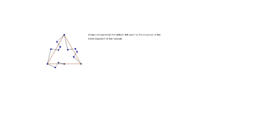Rotation Tessellation Triangle Step 8

Activity

EKaye

•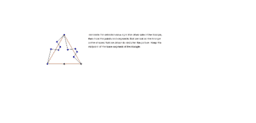Rotation Tessellation Triangle Step 7

Activity

EKaye

•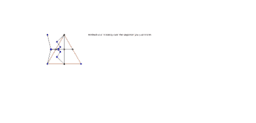Rotation Tessellation Triangle Step 6

Activity

EKaye

•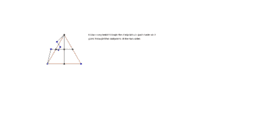Rotation Tessellation Triangle Step 5

Activity

EKaye

•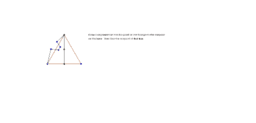Rotation Tessellation Triangle Step 4

Activity

EKaye

•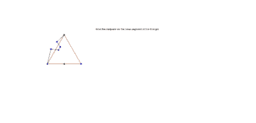Rotation Tessellation Triangle Step 3

Activity

EKaye

•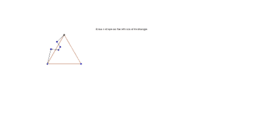Rotation Tessellation Triangle Step 2

Activity

EKaye

•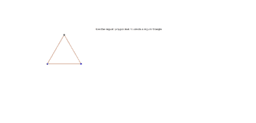Rotation Tessellation Triangle Step 1

Activity

EKaye

•Rotation Tessellation Triangle

Activity

EKaye

•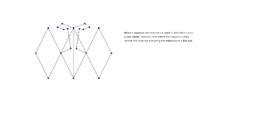Activity

EKaye